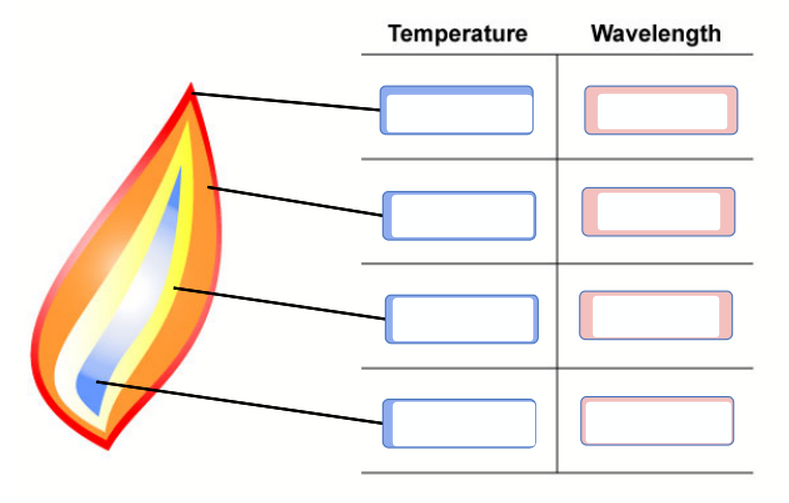# Problem: Part B. Using the visible region of the EM spectrum from the transition as a guide, label each indicated region of the hypothetical flame with the appropriate wavelength and temperature description.Coldest2nd coldest2nd hottesthottestlongest2nd longest2nd shortestshortestVisible light is a type of electromagnetic (EM) radiation. EM radiation has wavelike characteristics, which are periodic oscillations with a fixed distance between peaks (wavelength, λ) and a consistent number of cycles per second (frequency, ν). The interactions between matter and light are quantized in nature, which means specific packets, rather than a continuous gradient, of EM radiation are absorbed and emitted by atoms. The relationship between frequency (ν, Greek nu) and wavelength (λ, Greek lambda) can be described by the following equation:c = λνwhere c is the speed of light. The speed of light is a constant that is specified for each medium, and it has the value of 2.998 × 108 m/s in vacuum. Therefore, the frequency of light can be determined if the wavelength is known and vice versa.The phenomenon known as blackbody radiation is the temperature-dependent emission of EM radiation. At any given temperature, a broad range of wavelengths is being emitted by materials, and the wavelength at which the emission intensity of EM radiation is highest is denoted as λmax. Both λmax and the range of wavelengths shift with temperature, as can be observed in the simulation

###### FREE Expert Solution
88% (31 ratings)View Complete Written Solution
###### Problem Details

Part B. Using the visible region of the EM spectrum from the transition as a guide, label each indicated region of the hypothetical flame with the appropriate wavelength and temperature description.Coldest
2nd coldest
2nd hottest
hottest

longest
2nd longest
2nd shortest
shortest

Visible light is a type of electromagnetic (EM) radiation. EM radiation has wavelike characteristics, which are periodic oscillations with a fixed distance between peaks (wavelength, λ) and a consistent number of cycles per second (frequency, ν). The interactions between matter and light are quantized in nature, which means specific packets, rather than a continuous gradient, of EM radiation are absorbed and emitted by atoms. The relationship between frequency (ν, Greek nu) and wavelength (λ, Greek lambda) can be described by the following equation:

c = λν

where c is the speed of light. The speed of light is a constant that is specified for each medium, and it has the value of 2.998 × 108 m/s in vacuum. Therefore, the frequency of light can be determined if the wavelength is known and vice versa.

The phenomenon known as blackbody radiation is the temperature-dependent emission of EM radiation. At any given temperature, a broad range of wavelengths is being emitted by materials, and the wavelength at which the emission intensity of EM radiation is highest is denoted as λmax. Both λmax and the range of wavelengths shift with temperature, as can be observed in the simulation